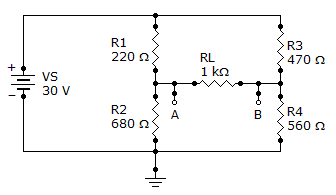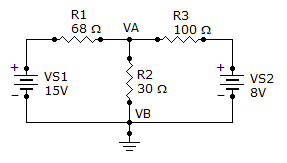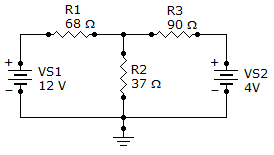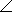# Online Electrical Engineering Test - Electrical Engineering Test 10Loading Test...

Instruction:

• This is a FREE online test. DO NOT pay money to anyone to attend this test.
• Total number of questions : 20.
• Time alloted : 30 minutes.
• Each question carry 1 mark, no negative marks.
• DO NOT refresh the page.
• All the best :-).

1.

Free electrons make current possible.

A.
 TrueB.
 False2.

If 750 µA is flowing through 11 kof resistance, what is the voltage drop across the resistor?

A.
 8.25 VB.
 82.5 VC.
 14.6 VD.
 146 V3.

At the end of a 14 day period, your utility bill shows that you have used 18 kWh. What is your average daily power?

A.
 1.286 kWhB.
 12.85 kWhC.
 535 kWhD.
 252 kWh4.

A half-watt is equal to how many milliwatts?

A.
 5,000 mWB.
 5 mWC.
 500 mWD.
 50 mW5.

Series aiding is a term sometimes used to describe voltage sources of the same polarity in series.

A.
 TrueB.
 False6.

If there are a total of 120 mA into a parallel circuit consisting of three branches, and two of the branch currents are 40 mA and 10 mA, the third branch current is

A.
 50 mAB.
 70 mAC.
 120 mAD.
 40 mA7.

In a parallel circuit, the branch with the lowest resistance has the most current.

A.
 TrueB.
 False8.

What is the Thevenin equivalent (VTH and RTH) for the circuit given?A.
 6.4 V, 422B.
 6.4 V, 560C.
 6.4 V, 680D.
 30 V, 4229.

What is the current through R2?A.
 3.19 AB.
 319 mAC.
 1.73 AD.
 173 mA10.

Find branch current IR2.A.
 5.4 mAB.
 –5.4 mAC.
 113.0 mAD.
 119.6 mA11.

Third-order determinants are evaluated by the expansion method or by the cofactor method.

A.
 TrueB.
 False12.

A 2F, a 4F, and a 10F capacitor are connected in series. The total capacitance is less than

A.
 2FB.
 4FC.
 10FD.
 1.5F13.

Five time constants are required for a capacitor to charge fully or discharge fully.

A.
 TrueB.
 False14.

In an RL circuit, the impedance is determined by both the resistance and the inductive reactance combined.

A.
 TrueB.
 False15.

A 10resistor, a 90 mH coil, and a 0.015F capacitor are in series across an ac source. The impedance magnitude at 1,200 Hz below fr is

A.
 1,616B.
 161C.
 3,387D.
 1,77116.

A certain series RLC circuit with a 200 Hz, 15 V ac source has the following values: R = 12, C = 80F, and L = 10 mH. The total impedance, expressed in polar form, is

A.
 12.2812.34°B.
 12.5712.34°C.
 9.9512.34°D.
 12.6212.34°17.

Referring to Problem 18, what is the bandwidth of the filter?

A.
 1 kHzB.
 632 kHzC.
 50.3 kHzD.
 10.5 kHz18.

In an RC differentiator, the sum of the capacitor voltage and the resistor voltage at any instant

A.
 must be zeroB.
 must be equal to the applied voltageC.
 is less than the applied voltage but greater than zeroD.
 cannot be determined19.

If the RC time constant of an integrator is increased, as the time constant is increased

A.
 the capacitor charges more during a pulse and discharges less between pulsesB.
 the capacitor charges less during a pulse and discharges more between pulsesC.
 the capacitor charges more during a pulse and discharges more between pulsesD.
 the capacitor charges less during a pulse and discharges less between pulses20.

In an RL integrating circuit, the output voltage is taken across the inductor.

A.
 TrueB.
 False